# Equation + polygon - math problems

#### Number of problems found: 9

• Polygon 42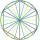Which polygon has 42 more diagonals than sides?
• Diagonals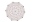What x-gon has 54 diagonals?
• Angles of a hexagon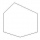Find the interior angles of a hexagon if the sizes of the angles form an arithmetic sequence and the smallest angle is 70°.
• Circle and hexagon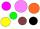Calculate the radius of a circle whose circumference is 8.4 cm longer than the circumference of the inscribed regular hexagon.
• Hexagon in circleCalculate the radius of a circle whose length is 10 cm greater than the circumference of a regular hexagon inscribed in this circle.
• Regular hexagonal prismCalculate the volume of a regular hexagonal prism whose body diagonals are 24cm and 25cm long.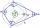What size have angles in quadrilateral (4-gon) if they are in a ratio of 8: 9: 10: 13?
• Hexagonal prism 2The regular hexagonal prism has a surface of 140 cm2 and height of 5 cm. Calculate its volume.
• n-gon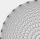Gabo draw n-gon, which angles are consecutive members of an arithmetic sequence. The smallest angle is 70° biggest 170°. How many sides have Gabo's n-gon?

We apologize, but in this category are not a lot of examples.
Do you have an interesting mathematical word problem that you can't solve it? Submit a math problem, and we can try to solve it.

We will send a solution to your e-mail address. Solved examples are also published here. Please enter the e-mail correctly and check whether you don't have a full mailbox.

Please do not submit problems from current active competitions such as Mathematical Olympiad, correspondence seminars etc...

Do you have a linear equation or system of equations and looking for its solution? Or do you have quadratic equation?